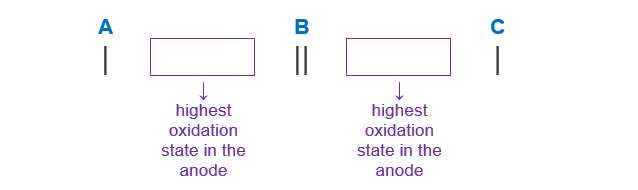# Problem: Determine the cell notation for the redox reaction given below. Sn(s) + 2 H+(aq) → Sn2+(aq) + H2(g) a) H2(g) | H+(aq) | Pt ll Sn2+(aq) | Sn(s) b) H+(aq) | H2(g) | Pt I| Sn(s)2+(aq)  c) Sn(s) | Sn2+(aq) || H+(aq) | H2(g) | Ptd) Sn(s) | H2g || Sn2+(aq) I H+(aq) | Pt e) Sn2+(aq) | Sn(s) || H2(g) | H+(aq) | Pt

###### FREE Expert SolutionSn (s)  Sn2+

Sn (s): O.S. = 0

Sn2+: O.S. = +2

change in OS: 0 to +2  increase/lose electrons  oxidation  anode

92% (171 ratings)###### Problem Details

Determine the cell notation for the redox reaction given below.

Sn(s) + 2 H+(aq) → Sn2+(aq) + H2(g)

a) H2(g) | H+(aq) | Pt ll Sn2+(aq) | Sn(s)

b) H+(aq) | H2(g) | Pt I| Sn(s)2+(aq)

c) Sn(s) | Sn2+(aq) || H+(aq) | H2(g) | Pt

d) Sn(s) | H2g || Sn2+(aq) I H+(aq) | Pt

e) Sn2+(aq) | Sn(s) || H2(g) | H+(aq) | Pt